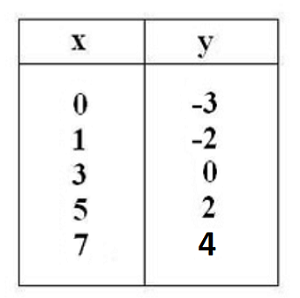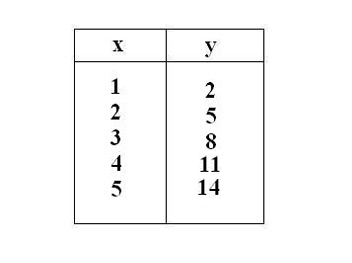# Algebraic Rule: Definition & Concept

Instructions:

Choose an answer and hit 'next'. You will receive your score and answers at the end.

question 1 of 3

### Write the algebraic rule for the following table of values.Create Your Account To Take This Quiz

As a member, you'll also get unlimited access to over 75,000 lessons in math, English, science, history, and more. Plus, get practice tests, quizzes, and personalized coaching to help you succeed.

Try it risk-free for 30 days. Cancel anytime.

### 1. Write the algebraic rule that supports the following data.### 2. Write the algebraic rule for the following, then solve. Your favorite store is having a sale. T-shirts cost \$5 and pants cost \$15. If you have \$100 to spend, and buy 3 pairs of pants, how many t-shirts can you buy?

Create your account to access this entire worksheet
A Premium account gives you access to all lesson, practice exams, quizzes & worksheets
Quizzes, practice exams & worksheets
Certificate of Completion
Create an account to get started

This quiz and worksheet will help you understand what algebraic rules are and how to create your own to define. Read on to learn more about translating multiple variables into a descriptive equation.

## Quiz & Worksheet Goals

With these resources, you can review basic principles of algebraic rules, such as the following:

• Creating algebraic rules from given information
• Using tables and graphs to discern pertinent rules
• Applying this knowledge to real-world scenarios

## Skills Practiced

• Problem solving - use acquired knowledge to solve for algebraic rules from given scenarios.
• Information recall - access the knowledge you've gained regarding how to create and map out rules in real life situations.
• Knowledge application - use your knowledge to create your own algebraic rules and solve the resulting equations.

Learn more about algebraic rules and their real-world applications. Review the accompanying lesson plan that covers the following objectives:

• Learn about algebraic rules that are commonly used, such as to calculate distance travelled or the area of an object.
• Examine different methods of constructing and defining algebraic rules.
• Understand how to apply basic algebra to solve problems faced in daily life.
Final ExamCAHSEE Math Exam: Tutoring Solution
Status: Not Started
Chapter ExamCAHSEE - Overview of Functions: Tutoring Solution
Status: Not Started

Support